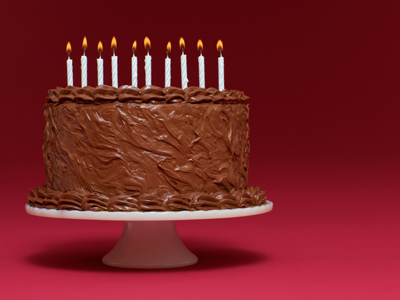How do you share a birthday cake equally?

# Fractions (Multiplying)

This Math quiz is called 'Fractions (Multiplying)' and it has been written by teachers to help you if you are studying the subject at middle school. Playing educational quizzes is a fabulous way to learn if you are in the 6th, 7th or 8th grade - aged 11 to 14.

It costs only \$12.50 per month to play this quiz and over 3,500 others that help you with your school work. You can subscribe on the page at Join Us

Do you remember what a fraction is? A fraction is a part of a whole number. For example, you have one birthday cake and you have ten people who each need an equal slice of that cake. The cake shall be cut into 10 equal slices and each slice (or part of the whole cake) will be equal to 110. That 110 is a fraction of the whole.

When adding and subtracting fractions you need to find a common denominator. Happily, in multiplying fractions you do not need to do that. In fact, many people find multiplying fractions easier than adding and subtracting.

For each multiplication problem below, find the correct solution/answer.

Go straight to Quiz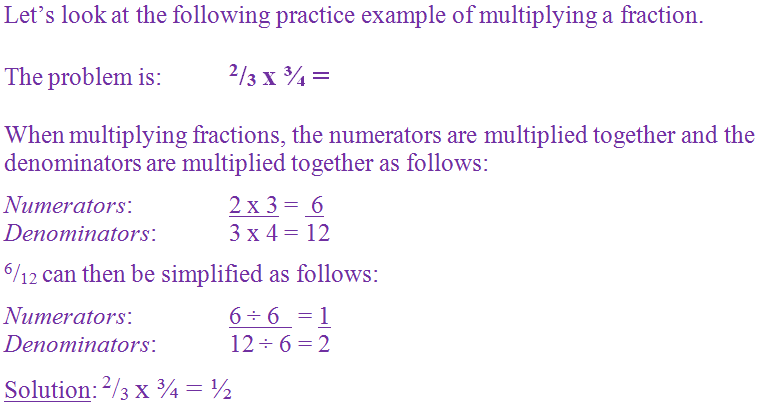1.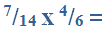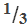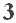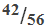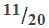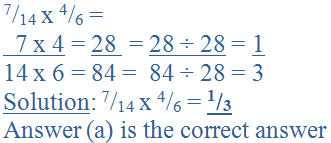2.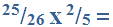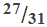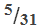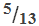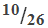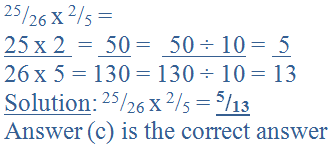3.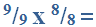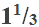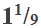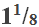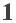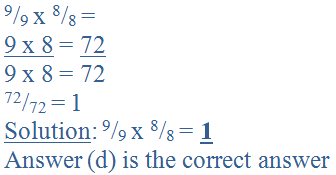4.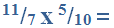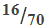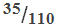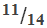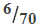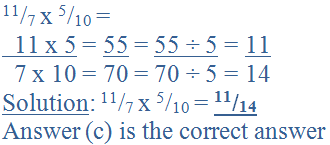5.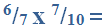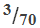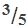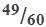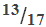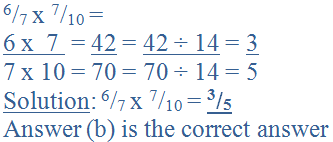6.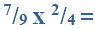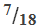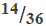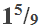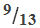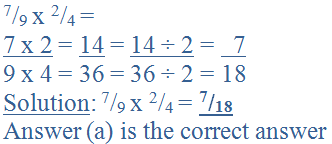7.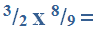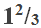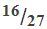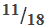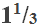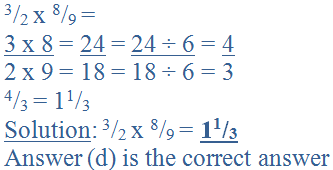8.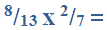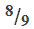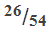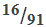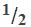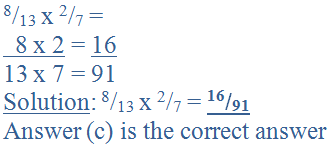9.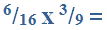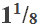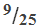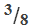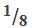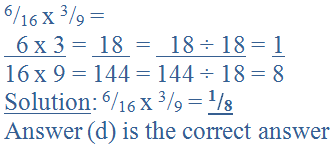10.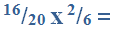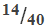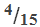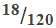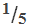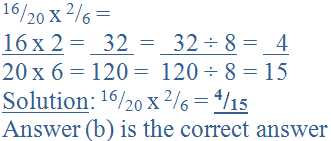Author:  Christine G. Broome